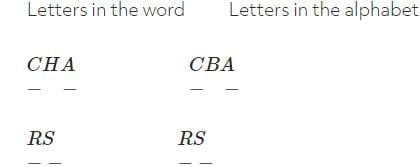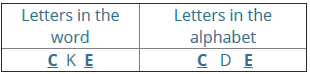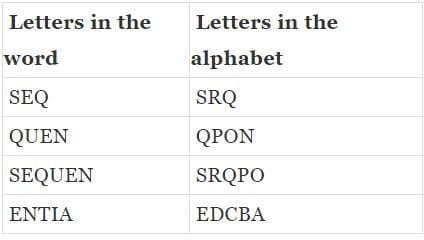CLAT  >  Test: Alphabet- 1

# Test: Alphabet- 1 - CLAT

Test Description

## 20 Questions MCQ Test Logical Reasoning for CLAT - Test: Alphabet- 1

Test: Alphabet- 1 for CLAT 2023 is part of Logical Reasoning for CLAT preparation. The Test: Alphabet- 1 questions and answers have been prepared according to the CLAT exam syllabus.The Test: Alphabet- 1 MCQs are made for CLAT 2023 Exam. Find important definitions, questions, notes, meanings, examples, exercises, MCQs and online tests for Test: Alphabet- 1 below.
Solutions of Test: Alphabet- 1 questions in English are available as part of our Logical Reasoning for CLAT for CLAT & Test: Alphabet- 1 solutions in Hindi for Logical Reasoning for CLAT course. Download more important topics, notes, lectures and mock test series for CLAT Exam by signing up for free. Attempt Test: Alphabet- 1 | 20 questions in 25 minutes | Mock test for CLAT preparation | Free important questions MCQ to study Logical Reasoning for CLAT for CLAT Exam | Download free PDF with solutions
 1 Crore+ students have signed up on EduRev. Have you?
Test: Alphabet- 1 - Question 1

### How many pairs of letters in the word 'CHAIRS' have as many letters between them in the word as in the alphabet?

Detailed Solution for Test: Alphabet- 1 - Question 1So Option A is correct

Test: Alphabet- 1 - Question 2

### Q: A B C D E F G H I J K L M N O P Q R S T U V W X Y Z. Which letter in this alphabet is the eighth letter to the right of the letter which is tenth letter to the left of the last but one letter of the alphabet?

Detailed Solution for Test: Alphabet- 1 - Question 2

In the given alphabet, last but one letter of alphabet is Y.

10th letter to the left of Y is O

8th letter to the right of O is W

Test: Alphabet- 1 - Question 3

### In the English Alphabet, which letter would be seventh to the left of fourteenth from right?

Detailed Solution for Test: Alphabet- 1 - Question 3

Fourteenth from right(Z to M) = M
Seventh from left of M is F.

Test: Alphabet- 1 - Question 4

In the English Alphabet, which letter would be tenth to the right of eighteenth from right?

Detailed Solution for Test: Alphabet- 1 - Question 4

Starting from right means Z to A.
When the alphabets are counted from backward the total number of alphabets is considered as 27.
Eighteenth from right is I = 27-18 = 9
The tenth alphabet to the right of I will be S = 9+10 = 19

Test: Alphabet- 1 - Question 5

In the English Alphabet, which letter would be 7th to the left of the letter S?

Detailed Solution for Test: Alphabet- 1 - Question 5

The number of S in alphabet series is 19
Seventh to left of S = L

Test: Alphabet- 1 - Question 6

If the first half of the English alphabet is reversed and then next portion of English alphabet is reversed so as 'A' takes the portion of 'M' and 'N' takes the portion of 'z' then which letter will be 6th to the left of 17th letter to the right of 7th leter from the left?

Detailed Solution for Test: Alphabet- 1 - Question 6

6th to the left of 17th letter to the right of 7th letter from the left = 6th to the left of (17+7 =) 24th letter from the left = (24-6 =) 18th letter from the left.
Thus, we have to count from the left and we have to find out 18th letter from the left that falls into the second section of English alphabet.
Here, both the first half and the second half of the English alphabet have been reversed and we have to count from the left end, then the required letter can be found out using the formula given below. Suppose, the first and the second reversed sections of the English alphabet are α and  β respectively.Then,
The required letter = 2α+β+1−(required position of letter from left side)
Therefore, The required letter = 26 + 13 + 1 - 18 = 22 = V

Test: Alphabet- 1 - Question 7

In the English Alphabet, which letter would be fifteenth to the right of letter which is third to the left of letter J?

Detailed Solution for Test: Alphabet- 1 - Question 7

When the alphabets are counted from backward the total number of alphabets is considered as 27.

The fifteeth letter to the right is 'L' according to 27 - 15 = 12 i.e. the 12th letter is 'L'.
The position of 'J' is 10 according to the English alphabet.
'L' position + 'J' position = 10 + 12 = 22 = V

Test: Alphabet- 1 - Question 8

In a certain code 'PENCIL' is written as 'RCTAMJ' then in that code 'BROKEN' is written as :

Detailed Solution for Test: Alphabet- 1 - Question 8

The first three letters of the word are reversed. Thus PENCIL becomes NEPCIL. Now add 4 to odd-positioned letters and subtract 2 from even-positioned ones.

Similarly, BROKEN becomes ORBKEN.
Then we do the calculations: O +4, R -2, B +4, K -2, E +4, N -2
Resulting word = SPFIIL

Test: Alphabet- 1 - Question 9

In the English Alphabet which letter would be 7th to the right of 15th from left?

Detailed Solution for Test: Alphabet- 1 - Question 9

Fifteen from left = O (15)
Seventh to right of O = 15+7 = 22
The 22th alphabet is V

Test: Alphabet- 1 - Question 10

If the second half of the english alphabet is written in reverse order, which letter will be ninth of the right of the eighth letter from the left end?

Detailed Solution for Test: Alphabet- 1 - Question 10

A,B,C,D,E,F,G,H,I,J,K,L,M,Z,Y,X,W,V,U,T,S,R,Q,P,O,N
Eighth letter from left end = H.
Ninth to the right of H = W.

Test: Alphabet- 1 - Question 11

If second half of the following alphabet is written in reverse order then which letter will be 10th from left of 9th letter from the right end?
A B C D E F G H I J K L M N O P Q R S T U V W X Y Z

Detailed Solution for Test: Alphabet- 1 - Question 11

A,B,C,D,E,F,G,H,I,J,K,L,M,Z,Y,X,W,V,U,T,S,R,Q,P,O,N
According to the arrangement
Ninth letter from the right end = V
Tenth letter to left of V = H.

Test: Alphabet- 1 - Question 12

Which letter is exactly midway between H and S in the alphabet series?

Detailed Solution for Test: Alphabet- 1 - Question 12

There are ten letters between H and S and as such there is no letter which lies in the middle

Test: Alphabet- 1 - Question 13

How many pairs of letters are there in the word BUCKET which has as many letters between them in the word as in the alphabet?

Detailed Solution for Test: Alphabet- 1 - Question 13Test: Alphabet- 1 - Question 14

Which of the following will be the changed form of the word OBLIQUE when the word is written again by substituting each vowel by the 2nd letter following it in the English alphabet and each consonant is substituted by the 3rd letter following it in the English alphabet?

Detailed Solution for Test: Alphabet- 1 - Question 14

The Given word is OBLIQUE.

After making necessary changes that are all vowels are substituted by the next second letter and all consonants are substituted by the next third letter in the English alphabet.

Thus, the resulting word is QEOKTWG.

Test: Alphabet- 1 - Question 15

If a meaningful word can be formed using the five letters NWROD each only once, then the fourth letter of that word is your answer. If more than one such word can be formed then Y is your answer, and if no such word can be formed then 'Z' is your answer.

Detailed Solution for Test: Alphabet- 1 - Question 15

Here specified letters are N,W,R,O and D. The meaningful word made from these letters is DROWN.
Thus, the answer is W the fourth letter of the only word possible.

Test: Alphabet- 1 - Question 16

If it is possible to make a meaningful word with the first, the fourth, the seventh and the eleventh letters of the word "INTERPRETATION', which of the following will be third letter of that word ? If more than one such word can be made, give M as the answer and if no such word can be made, give X as the answer.

Detailed Solution for Test: Alphabet- 1 - Question 16

The first, fourth, seventh and eleventh letters of the word INTERPRETATION are I, E, R and T respectively. The words formed are TIER, RITE and TIRE.

Thus M is the answer as more than one word can be formed.

Test: Alphabet- 1 - Question 17

If the alphabet is written in the reverse order and every alternate letter starting with Y is dropped, which letter will be exactly in the middle of the remaining, letters of the alphabet.

Detailed Solution for Test: Alphabet- 1 - Question 17

Canceling every second letter after reversing the alphabet the series becomes: Z X V T R P N L J H F D B
Thus, the middle letter is N

Test: Alphabet- 1 - Question 18

If it is possible to make a meaningful word with the 2nd, the 3rd, the 4th and the 6th letter of the word STRATEGIC; then which of the following will be the 2nd letter of the word? If no such word can be made, mark ‘X’ as your answer. If more than one such word can be made, mark ‘M’ as your answer.

Detailed Solution for Test: Alphabet- 1 - Question 18

The 2nd, the 3rd, the 4th and the 6th letter of the word STRATEGIC are T,R,A and E respectively.
The words can be RATE and TEAR.
Thus M is the answer as more than one word can be formed.

Test: Alphabet- 1 - Question 19

A B C D E F G H I J K L M N O P Q R S T U V W X Y Z

If the first half of the above alphabets are written in the reverse order, which letter will be exactly middle between the 9th letter from the left and the 10th letter from the right end?

Detailed Solution for Test: Alphabet- 1 - Question 19

The arrangement will be: M L K J I H G F E D C B A N O P Q R S T U V W X Y Z
9th letter from left = E.
10th letter from right = Q
A lies exactly in middle between E and Q

Test: Alphabet- 1 - Question 20

How many pairs of letters are there in the word 'SEQUENTIAL' which have as many letters between them as are in the alphabet?

Detailed Solution for Test: Alphabet- 1 - Question 20## Logical Reasoning for CLAT

19 videos|27 docs|67 tests
Information about Test: Alphabet- 1 Page
In this test you can find the Exam questions for Test: Alphabet- 1 solved & explained in the simplest way possible. Besides giving Questions and answers for Test: Alphabet- 1, EduRev gives you an ample number of Online tests for practice

## Logical Reasoning for CLAT

19 videos|27 docs|67 tests(Scan QR code)